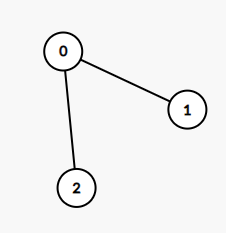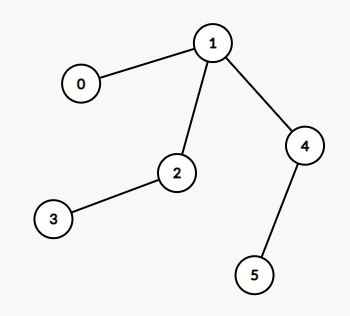##### Welcome to Subscribe On Youtube

Formatted question description: https://leetcode.ca/all/1245.html

# 1245. Tree Diameter

Medium

## Description

Given an undirected tree, return its diameter: the number of edges in a longest path in that tree.

The tree is given as an array of edges where edges[i] = [u, v] is a bidirectional edge between nodes u and v. Each node has labels in the set {0, 1, ..., edges.length}.

Example 1:Input: edges = [[0,1],[0,2]]

Output: 2

Explanation:

A longest path of the tree is the path 1 - 0 - 2.

Example 2:Input: edges = [[0,1],[1,2],[2,3],[1,4],[4,5]]

Output: 4

Explanation:

A longest path of the tree is the path 3 - 2 - 1 - 4 - 5.

Constraints:

• 0 <= edges.length < 10^4
• edges[i] != edges[i]
• 0 <= edges[i][j] <= edges.length
• The given edges form an undirected tree.

## Solution

For each node, obtain its number of degrees, which is the number of nodes that the node directly connects to, and all the neighbors of the node.

Do breadth first search starting from the nodes with degree 1. Each time a node is visited, decrease its degree by 1, obtain all its neighbors, and decrease each neighbor’s degree by 1. If a neighbor’s degree becomes 1, then offer the neighbor to the queue for the next step’s search. During the search, maintain the depth of the search.

Finally, there will be 1 node or 2 nodes remaining. If there is 1 node remaining, then the diameter is twice the depth. If there are 2 nodes remaining, then there is an edge that connects the 2 nodes, so the diameter is twice the depth plus 1.

• class Solution {
public int treeDiameter(int[][] edges) {
int nodes = edges.length + 1;
int[] degrees = new int[nodes];
Set<Integer>[] connects = new Set[nodes];
for (int i = 0; i < nodes; i++)
connects[i] = new HashSet<Integer>();
for (int[] edge : edges) {
int node1 = edge, node2 = edge;
degrees[node1]++;
degrees[node2]++;
}
for (int i = 0; i < nodes; i++) {
if (degrees[i] == 1)
queue.offer(i);
}
int depth = 0;
int remaining = nodes;
while (!queue.isEmpty() && remaining > 2) {
int size = queue.size();
for (int i = 0; i < size; i++) {
int node = queue.poll();
degrees[node]--;
Set<Integer> connectSet = connects[node];
for (int connect : connectSet) {
degrees[connect]--;
if (degrees[connect] == 1)
queue.offer(connect);
}
}
remaining -= size;
depth++;
}
return remaining == 2 ? 2 * depth + 1 : 2 * depth;
}
}

• // OJ: https://leetcode.com/problems/tree-diameter/
// Time: O(N)
// Space: O(N)
class Solution {
public:
int treeDiameter(vector<vector<int>>& E) {
int N = E.size() + 1, step = 0;
vector<vector<int>> G(N);
vector<int> indegree(N);
for (auto &e : E) {
G[e].push_back(e);
G[e].push_back(e);
indegree[e]++;
indegree[e]++;
}
queue<int> q;
for (int i = 0; i < N; ++i) {
if (indegree[i] == 1) q.push(i);
}
while (q.size() > 1) {
int cnt = q.size();
while (cnt--) {
int u = q.front();
q.pop();
--indegree[u];
for (int v : G[u]) {
if (indegree[v] == 0) continue;
if (--indegree[v] == 1) {
q.push(v);
}
}
}
++step;
}
return step * 2 + q.size() - 1;
}
};

• class Solution:
def treeDiameter(self, edges: List[List[int]]) -> int:
def dfs(u, t):
nonlocal ans, vis, d, next
if vis[u]:
return
vis[u] = True
for v in d[u]:
dfs(v, t + 1)
if ans < t:
ans = t
next = u

d = defaultdict(set)
vis = [False] * (len(edges) + 1)
for u, v in edges:
ans = 0
next = 0
dfs(edges, 0)
vis = [False] * (len(edges) + 1)
dfs(next, 0)
return ans


• func treeDiameter(edges [][]int) int {
n := len(edges)
g := make(map[int][]int)
for _, e := range edges {
g[e] = append(g[e], e)
g[e] = append(g[e], e)
}
vis := make(map[int]bool, n+1)
ans := 0
next := edges
var dfs func(u, t int)
dfs = func(u, t int) {
if vis[u] {
return
}
vis[u] = true
if ans < t {
ans = t
next = u
}
if vs, ok := g[u]; ok {
for _, v := range vs {
dfs(v, t+1)
}
}
}
dfs(next, 0)
vis = make(map[int]bool, n+1)
dfs(next, 0)
return ans
}